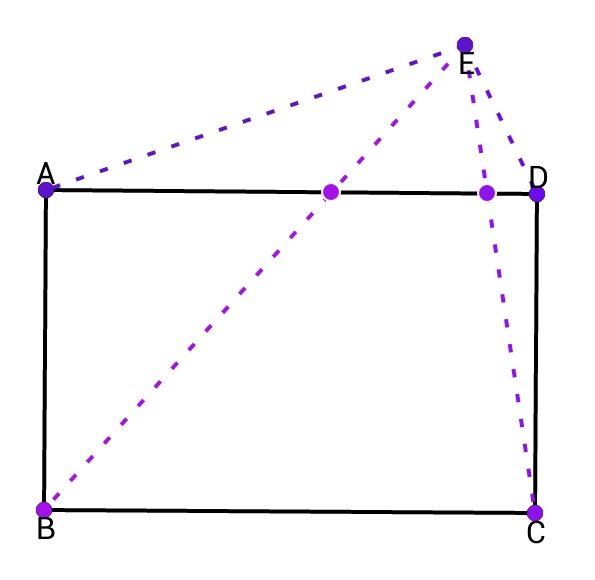# Rectangle & a Point outside it

Geometry Level 3$ABCD$ is a rectangle such that $AB:BC=3:2$. There's a point $E$ in its plane such that, $\alpha EA^2+\beta EB^2+\gamma EC^2+\delta ED^2=0$. Find $\alpha+\beta+\gamma+\delta$

×